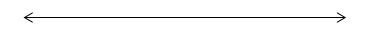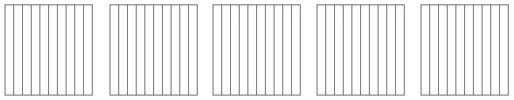# Decimal Fractions

## Objective

Represent decimals to tenths less than ten with number lines. Convert between fraction, decimal, and unit form.

## Common Core Standards

### Core Standards

?

• 4.NF.C.6 — Use decimal notation for fractions with denominators 10 or 100. For example, rewrite 0.62 as 62/100; describe a length as 0.62 meters; locate 0.62 on a number line diagram.

?

• 3.NF.A.2

## Criteria for Success

?

1. Represent decimals to tenths less than ten written in decimal, fraction, or unit form using an area model (MP.5).
2. Represent decimals to tenths less than ten written in decimal, fraction, or unit form using a number line (MP.5).
3. Convert between fraction form, decimal form, and unit form for some number of tenths less than ten.
4. Understand that 1 one can be regrouped as 10 tenths and flexibly write numbers in fraction and unit form based on that understanding.

## Tips for Teachers

?

• Some resources find that expanded form seems more aligned to 5.NBT.1, given that’s the first place it is explicitly mentioned in the Common Core State Standards in relation to decimals. However, given that expanded form deepens students’ understanding of the value of each digit in a decimal and there are reliable sources such as this Illustrative Mathematics task Expanded Fractions and Decimals that include it in Grade 4, it has been included throughout this unit. However, it is at your discretion to cut it.
• When saying a number in word form, make sure to only use the word “and” in place of the decimal place and nowhere else. For example, 7.5 is read “seven and five tenths.”

#### Fishtank Plus

• Problem Set
• Student Handout Editor
• Vocabulary Package

?

### Problem 1

Fill in the table below based on the location of each point on the number line.Point Decimal Form Fraction Form Unit Form A B C D E

### Problem 2

Danny is converting a number between decimal, fraction, and unit form. He wrote the following:

 Decimal Form Fraction Form Unit Form 2.4 $\frac{24}{10}$ 24 tenths

Do you agree or disagree with Danny’s reasoning? Why or why not? Use a picture to support your reasoning.

## Discussion of Problem Set

?

• Look at #1b. How many tenths did you shade in total? How do you know?
• Look at #1e. How did you know how much of the rectangles to shade in? What is the most efficient way to determine how many rectangles you would need to shade in?
• Look at #2. What endpoints did you choose to plot each number? Could you have chosen other ones?
• Look at #3. For the third row in the chart, I wrote the decimal 3.4. Do you agree or disagree with my answer? Why?

?

### Problem 1

Place the decimal below on a number line. Express the decimal as an equivalent mixed number.

4.8#### References

EngageNY Mathematics Grade 4 Mathematics > Module 6 > Topic A > Lesson 2Exit Ticket, Question #1

Grade 4 Mathematics > Module 6 > Topic A > Lesson 2 of the New York State Common Core Mathematics Curriculum from EngageNY and Great Minds. © 2015 Great Minds. Licensed by EngageNY of the New York State Education Department under the CC BY-NC-SA 3.0 US license. Accessed Dec. 2, 2016, 5:15 p.m..

Modified by The Match Foundation, Inc.

### Problem 2

Write the following in decimal form, unit form, and fraction form. Shade the area model to match.

a.  3 ones and 7 tenths = ________ = ________b.  ${2{4\over10}}$ = ________ = ________#### References

EngageNY Mathematics Grade 4 Mathematics > Module 6 > Topic A > Lesson 2Exit Ticket, Question #2

Grade 4 Mathematics > Module 6 > Topic A > Lesson 2 of the New York State Common Core Mathematics Curriculum from EngageNY and Great Minds. © 2015 Great Minds. Licensed by EngageNY of the New York State Education Department under the CC BY-NC-SA 3.0 US license. Accessed Dec. 2, 2016, 5:15 p.m..

Modified by The Match Foundation, Inc.

?